• 主要为大家详细介绍了利用OpenCV实现局部动态阈值分割，具有一定的参考价值，感兴趣的小伙伴们可以参考一下
• Local_Yanowitz 由于光照的影响，图像的灰度可能是不均匀分布的，此时...Yanowitz提出了一种局部阈值分割方法。结合边缘和灰度信息找到阈值表面（treshhold surface）。在阈值表面上的就是目标。 算法的主要...
github地址：https://github.com/radishgiant/ThresholdAndSegment.git
Local_Yanowitz
由于光照的影响，图像的灰度可能是不均匀分布的，此时单一阈值的方法分割效果不好。Yanowitz提出了一种局部阈值分割方法。结合边缘和灰度信息找到阈值表面（treshhold surface）。在阈值表面上的就是目标。算法的主要步骤如下：  - step1：均值平滑图像  - step2：求平滑图像的梯度图  - step3：运用Laplacian算子，找到具有局部最大阈值的点，这些点的原始灰度值就是候选的局部阈值。- step4 ：采样候选点，灰度值替换。将平滑图像中的候选点灰度值替换为原始图像中的灰度值或者更大一点的值。这么做的目的是不会检测到虚假目标，因而会损失一部分真实的目标。  - step5：插值灰度点，得到阈值表面。

limn→∞Pn(x,y)=Pn−1(x,y)+β⋅Rn(x,y)4

l

i

m

n

→

∞

P

n

(

x

,

y

)

=

P

n

−

1

(

x

,

y

)

+

β

⋅

R

n

(

x

,

y

)

4

lim_{n \to \infty}P_n(x,y)=P_{n-1}(x,y)+\frac{\beta\cdot R_n(x,y)}{4}

R(x,y)=P(x,y+1)+P(x,y−1)+P(x−1,y)+P(x+1,y)−4P(x,y)

R

(

x

,

y

)

=

P

(

x

,

y

+

1

)

+

P

(

x

,

y

−

1

)

+

P

(

x

−

1

,

y

)

+

P

(

x

+

1

,

y

)

−

4

P

(

x

,

y

)

R(x,y)=P(x,y+1)+P(x,y-1)+P(x-1,y)+P(x+1,y)-4P(x,y)

其中，只有当

β=0

β

=

0

$\beta=0$时，残差消失(residual vanish)。

1<β<2

1

<

β

<

2

$1< \beta <2$时收敛更快。

R(x,y)

R

(

x

,

y

)

$R(x,y)$为拉普拉斯算子，强迫任意点

R(x,y)=0

R

(

x

,

y

)

=

0

$R(x,y)=0$的几何意义是使得曲线光滑。光滑曲线的梯度是连续变化的，因而其二次导数为0。
- step6：阈值表面分割图像
- step7：校正。由于光照和噪声，阈值表面和原始原始灰度曲线可能相交如下图所示。可以看到分割结果中出现 “ghost” 目标，应该予以去除。去除的原理是，这些虚假的目标边缘梯度值应该较小。因而，可以根据分割的结果，标记所有连通区域，注意背景和目标应该分开标记。比较标记部分边缘在梯度图中的值，如果某个目标的边缘梯度的平均值不超过某个阈值，则去除这个目标。matlab 代码
function [P_new,label]=Local_Yanowitz(I,varargin);
% local adaptive  treshold segment by Yanowitz
% input:
%       I is Image M by N
%reference:S. D. Yanowitz and A. M. Bruckstein, "A new method for image
% segmentation," Comput. Graph. Image Process. 46, 82–95 ,1989.
dbstop if error
if nargin<=1||isempty(varargin{1});
hsize=[3,3];
end
if nargin<=2||isempty(varargin{2});
MaxInterNum=15500;
end
if nargin<=3||isempty(varargin{3});
InterTreshhold=10e-6;
end
if nargin<=4||isempty(varargin{4});
end
% I=double(I);
[M,N]=size(I);
% step1:smooth the image
h1=fspecial('average',hsize);
SI=imfilter(I,h1);
F=sqrt(Fx.^2+Fy.^2);
%step3 :Laplacian Image
h2=fspecial('laplacian',0);
LI=imfilter(SI,h2);
%step4:sample the smoothed image at the places which the maximal
P_new=zeros(M,N);
P_new(LI==0)=I(LI==0);

% step5: interpolate the sampled gray level over the image
Residual=InterTreshhold+1;
InterNum=0;
while (Residual>InterTreshhold)
if(InterNum>MaxInterNum)
fprintf('up to MaxInterNum without diveregence');
break;
end
InterNum=InterNum+1;
P_last=P_new;
R=imfilter(P_new,h2);
P_new=P_new+R./4;
Residual=mean(abs(P_new(:)-P_last(:)));

end

% step:6 segment the Image
bw=zeros(M,N);
bw(I>P_new)=255;%background
figure,imshow(bw);title('first segment result')
% step:7 validation progress
label=bwlabel(bw,4);
RGBLabel=label2rgb(label);
figure,imshow(RGBLabel);title('connected component');
lable_n=length(unique(label));
toglabel=zeros(lable_n,1);
for ci=0:lable_n-1
temp=zeros(size(I));
temp(label==ci)=255;
eg=edge(temp);
[egr,egc]=find(eg==1);
[~,mingI]=min(F(eg>=1));% find the location of gradient of min value in eg
mingr=egr(mingI);%find the location of gradient of min value  over image
mingc=egc(mingI);

nearborlabel=[mingr+1,mingc;mingr-1,mingc;mingr,mingc+1;mingr,mingc-1];
nearborlogical=ones(4,1);
if (mingr==1)
nearborlogical(2)=0;
end
if (mingr==M)
nearborlogical(1)=0;
end
if (mingc==1)
nearborlogical(4)=0;
end
if mingc==N
nearborlogical(3)=0;
end
nearborlabel=nearborlabel(nearborlogical==1,:);
nearborlabel=label(sub2ind([M,N],nearborlabel(:,1),nearborlabel(:,2)));
dlilabel=label(mingr,mingc);
if nnz(nearborlabel~=dlilabel)
toglabel(ci+1)=mode(nearborlabel(nearborlabel~=dlilabel));
else
toglabel(ci+1)=dlilabel;
end
end
% find background label
bl=mode(label(bw==255));
for di=1:length(dli)

label(label==dli(di))=toglabel(dli(di));
end
RGBLabel=label2rgb(label);
figure,imshow(RGBLabel);title('segment result after valiation');
figure,plot(1:N,I(mingr,:),1:N,P_new(mingr,:));
legend('gray level','Treshold surface');
end

参考文献
S. D. Yanowitz and A. M. Bruckstein, “A new method for image  segmentation,” Comput. Graph. Image Process. 46, 82–95 ,1989.
展开全文• 基于Niblack's method方法的局部阈值分割 Email:whowhoha@outlook.com  /*原理：   T(x,y)=m(x,y) + k*s(x,y)   取一个宽度为w的矩形框，(x,y)为这个框的中心。  统计框内数据，T(x,y)为阈值,m(x...
基于Niblack's method方法的局部阈值分割

Email:whowhoha@outlook.com

/*原理：            T(x,y)=m(x,y)  +   k*s(x,y)            取一个宽度为w的矩形框，(x,y)为这个框的中心。         统计框内数据，T(x,y)为阈值,m(x,y)为均值，s(x,y)为均方差，k为参数(推荐-2)计算出t再对(x,y)进行切割255/0。            这个算法的优点是    速度快，效果好。            缺点是    niblack's   method会产生一定的噪声。       */          public int[]localThresholdProcess(int []data,int width,intheight,int w,int h,doublecoefficients,double gate){     int[] processData=newint[data.length];     for(inti=0;i<data.length;i++){       processData[i]=255;     }          if(data.length!=width*height)        returnprocessData;          int wNum=width/w;     int hNum=height/h;     int delt[]=new int[w*h];          //System.out.println("w;"+w+"h:"+h+" wNum:"+wNum+" hNum:"+hNum);          for(int j=0;j<hNum;j++){        for(inti=0;i<wNum;i++){     //for(int j=0;j<1;j++){     // for(int i=0;i<1;i++){          for(intn=0;n<h;n++)              for(intk=0;k<w;k++){              delt[n*w+k]=data[(j*h+n)*width+i*w+k];         &nbsp;   //System.out.print("delt["+(n*w+k)+"]:"+delt[n*w+k]+"");              }        //System.out.println();         /*         for(intn=0;n<h;n++)              for(intk=0;k<w;k++){              System.out.print("data["+((j*h+n)*width+i*w+k)+"]:"+data[(j*h+n)*width+i*w+k]+"");              }        System.out.println();         */        delt=thresholdProcess(delt,w,h,coefficients,gate);         for(intn=0;n<h;n++)              for(intk=0;k<w;k++){              processData[(j*h+n)*width+i*w+k]=delt[n*w+k];              //System.out.print("delt["+(n*w+k)+"]:"+delt[n*w+k]+"");              }        //System.out.println();         /*         for(intn=0;n<h;n++)              for(intk=0;k<w;k++){              System.out.print("processData["+((j*h+n)*width+i*w+k)+"]:"+processData[(j*h+n)*width+i*w+k]+"");              }        System.out.println();         */        }      }          return processData;     }
展开全文二值化
• 全局阈值分割
全局阈值分割
迭代法阈值获取方法： 1）选取一个的初始估计值T（128)； 2）用T分割图像。这样便会生成两组像素集合：G1由所有灰度值大于T的像素组成，而G2由所有灰度值小于或等于T的像素组成。 3）对G1和G2中所有像素计算平均灰度值u1和u2。 4）计算新的阈值：T=1/2(u1 + u2)。 重复步骤（2）到（4），直到得到的T值之差小于一个事先定义的参数T0。
先导进来图片
I = imread('rice.png');
[width,height] = size(I);
T0 = 1;%设置门限
T1= 128; %初始阈值T1
%设置G1，G2两个列向量，各自统计<T和>T的值
gray_leval_1 = 1;
gray_leval_2 = 1;

matlab里没有do-while语句，所以用 while 1 … break 代替
while 1
for i = 1:width
for j = 1:height
if I(i,j)>T1
G1(gray_leval_1) = I(i,j); %得到分组G1
gray_leval_1 = gray_leval_1 + 1;
else
G2(gray_leval_2) = I(i,j); %得到分组G2
gray_leval_2 = gray_leval_2 + 1;
end
end
end
%计算G1、G2均值
avg1 = mean(G1);
avg2 = mean(G2);
T2 = (avg1 + avg2)/2;
if abs(T2 - T1)<T0
break;
end
T1 = T2;
gray_leval_1 = 1;
gray_leval_2 = 1;
end
T1
T1=uint8(T1);

T1=130.7 然后画出来
for i=1:width
for j=1:height
if(I(i,j)<T1)
BW1(i,j)=0;
else
BW1(i,j)=1;
end
end
end
subplot(1,3,1);imshow(I)
subplot(1,3,2);imshow(BW1);title('阈值分割');

matlab自带的Otus阈值分割方法
T3=graythresh(I)
BW2=imbinarize(I,T3);%Otus阈值分割
subplot(1,3,3);imshow(BW2);title('Otus阈值分割');

I=130.9,差不多哈。 结果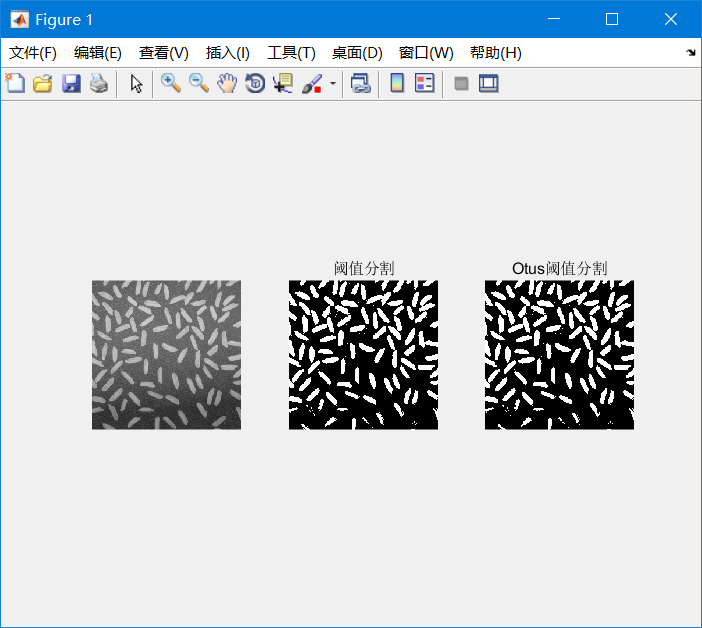这个阈值分割方法存在偏差，如果两个峰值数目相差越多，偏差越大 （假设第一次迭代前，T在正确位置，很容易看出来，随着迭代进行，越来越偏离）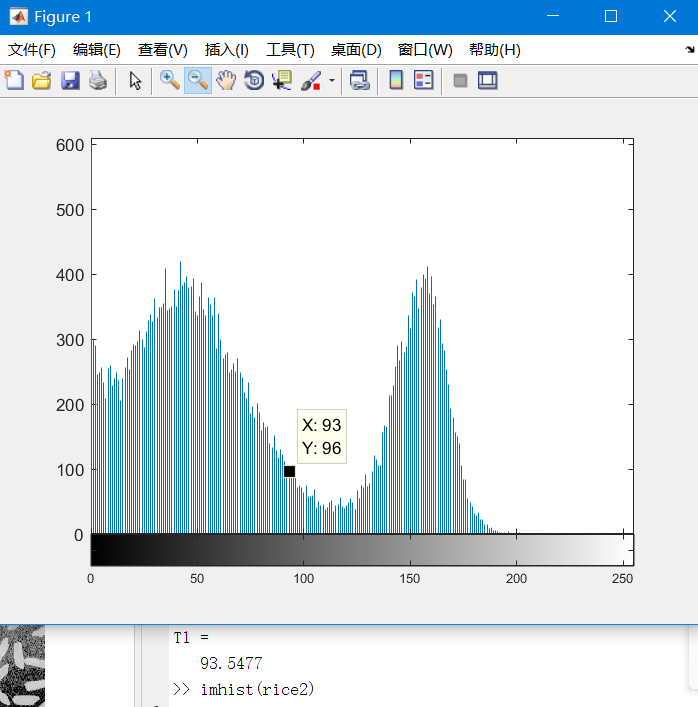计算的T=93，目测值应该在110-120吧。
多说一句，图像处理，一定要double一下，否则可能出现问题。比如，我想把大米图的直方图暗部拉大（偏向0），以体现这种偏差，就用了个一次函数的变换，结果这样了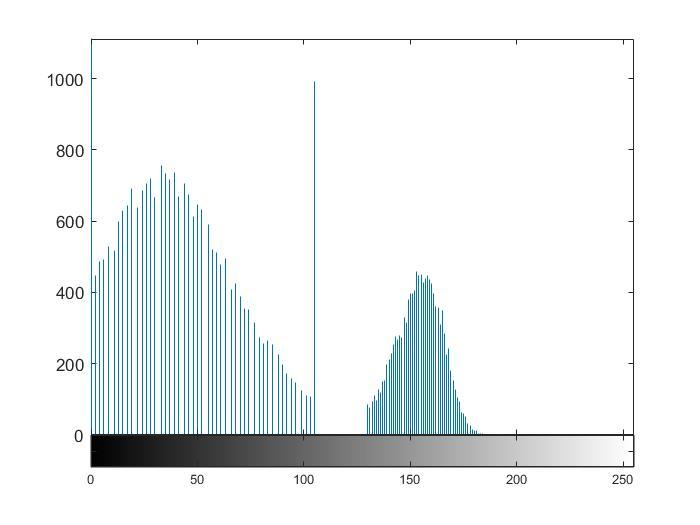因为先乘k，结果>255的全归于255，以后全错。
自适应阈值分割
1）将整幅图像分成一系列互相之间有50%重叠的子图像； 2）做出每个子图像的直方图； 3）检测各个子图像的直方图是否为双峰，如果是，则采用最佳阈值法确定一个阈值，否则就不进行处理； 4）根据对直方图为双峰的子图像得到的阈值通过插值得到所有子图像（像素点）的阈值。 仨坑 1、怎么割图像啊（这个还好吧，也不算割，设置好步长来就行了） 2、怎么检测单峰还是双峰啊，单峰的话，空缺imresize是不行的。 3、怎么插值啊（主要是老师上课讲的太玄学，按照距离加权，四点平均，把相距很远的点内部全部插满，听懵了。后来想到，matlab直接imresize啊！然后就码下来啦） 1、分割方式设置好矩形大小和步长就行 2、峰值个数没写，matlab统计工具箱里好像有正态拟合图像，而不是plot（）这种拟合，plot拟合只是画的圆滑了些还是很多局部峰值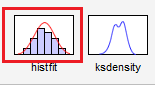3、插值方式采用了imresize函数 A=imresize(I,2,‘nearest’);%最近邻插值 B=imresize(I,2,‘bilinear’);%双线性 C=imresize(I,2,‘bicubic’);%双三次
I=imread('rice.png');
T=zeros(15);
for i=0:14
for j=0:14
img = imcrop(I,[1+i*16 1+j*16 31 31])；%内核32*32，步长设置为16
t=255*graythresh(img);%我这里直接用了
T(j+1,i+1)=t;%这里注意坐标顺序反着
end
end
T=uint8(T);
T1=imresize(T,[256 256],'bilinear');%看了下貌似双线性插值最好
%%
for i=1:256
for j=1:256
if (I(i,j)<T1(i,j))
BW(i,j)=0;
else
BW(i,j)=1;
end
end
end
imshow(BW)

有一个地方要注意，图像[i,j],矩阵得是[j,i]。
阈值图像：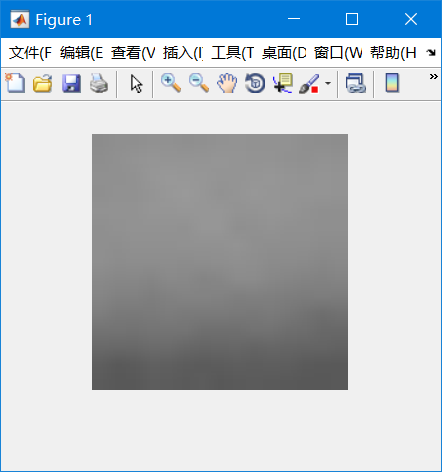底部更暗，所以阈值也更低才能滤完整 阈值分割结果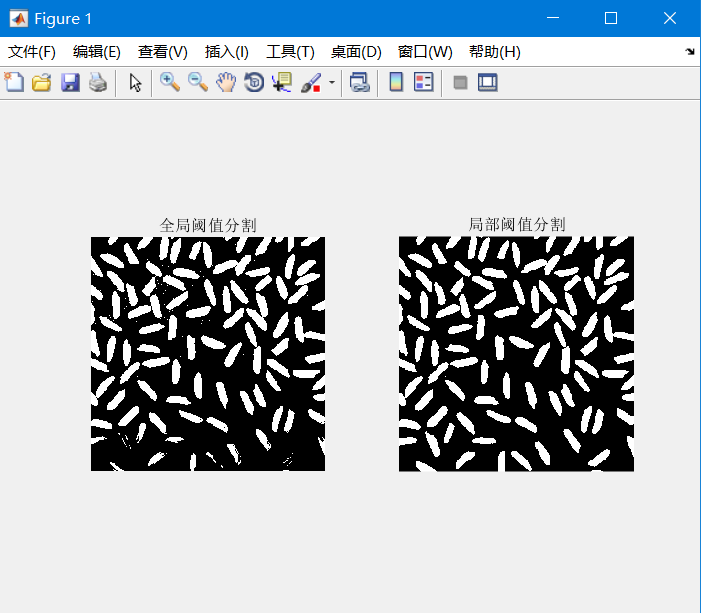嗯，上部噪声少了，下部也更完整了 然后试了下这位兄弟博客的图片 这老哥跑的OPENCV中自带的函数，效果一级棒（怎么看着像边缘检测） 我这个…毕竟没字的地方有黑背景有白背景，一加权，也没法搞，不过还阔以吧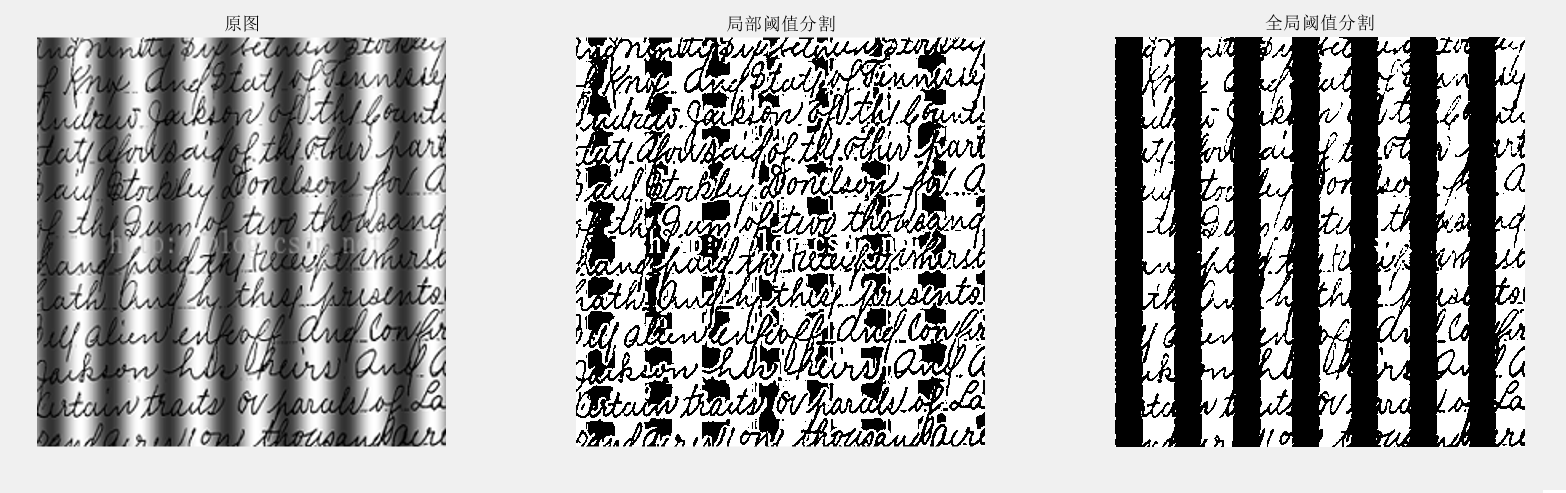matlab中自带的阈值分割，对大米图还行。全局阈值分割底部大米都割残了，这个方法完整，可是，这跟局部有关系？貌似先调了调灰度，就好分割了
 I=imread('rice.png');
I=im2double(I);
se=strel('disk',10);%se.Neighborhood:10*10圆盘
ft=imtophat(I,se);% 高帽变换
gt=uint8(255*ft);
Th=graythresh(ft);
G=imbinarize(ft,Th);%阈值分割
figure,imshow(G),title('局部阈值');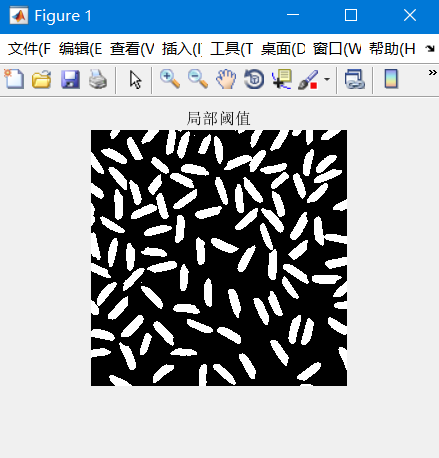Otsu算法
matlab自带的阈值分割方法 参考动态阈值–大津法和基于Otsu算法的图像自适应阈值分割，重新打了一下 调用：

[counts x] = imhist(img); [m n] = size(img); BW1 = otsu(counts, m*n); 第一个元素为直方图，第二个元素为图片大小 或者： T=graythresh(I) BW2=imbinarize(I,T);

原理：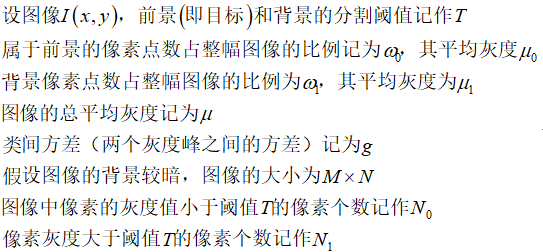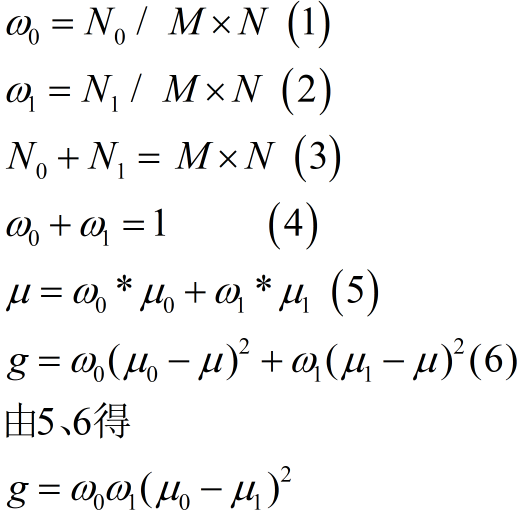让T遍历所有灰度值，找到g的最大值 代码：
function level = otsu(histogramCounts, total)%histogramCounts是图像的直方图, total图像的总像素数
sum0 = 0;
w0 = 0;%像素个数
maximum = 0.0;%保存T的最大值
total_value = sum((0:255).*histogramCounts');
for ii=1:256
w0 = w0 + histogramCounts(ii);%<T像素个数累加
if (w0 == 0)
continue;
end
w1 = total - w0;
if (w1 == 0)%遍历结束>T像素=0
break;
end
sum0 = sum0 +  (ii-1) * histogramCounts(ii);%灰度和
m0 = sum0 / w0;%平局灰度u0
m1 = (total_value - sum0) / w1;%平局灰度u1
icv = w0 * w1 * (m0 - m1) * (m0 - m1);
if ( icv >= maximum )
level = ii;
maximum = icv;
end
end
end

展开全文• 不过话说回来人能分辨出来的东西代码也能，只不过怎么把原理提取出来。 今日在网上找到一案例： 好图： 坏图： 要求是检测右边的那些斑点。看似非常简单，立马就产生了思路，写的时候猛的发现这个视觉系统的...
在背景较差的表面进行任何一种检测都是非常不理想的。不过话说回来人能分辨出来的东西代码也能，只不过怎么把原理提取出来。
今日在网上找到一案例：
好图：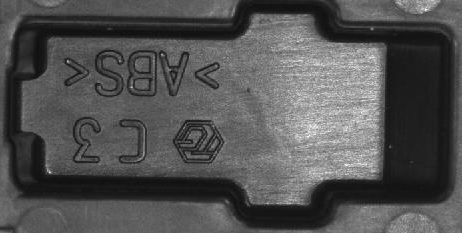坏图：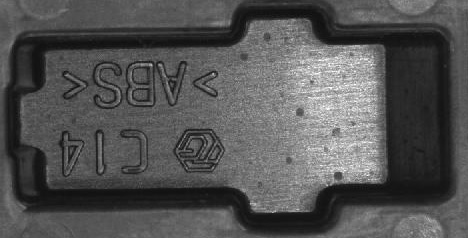要求是检测右边的那些斑点。看似非常简单，立马就产生了思路，写的时候猛的发现这个视觉系统的打光不好，有强暗反射的偏差，以及它的背景影响太大。那么前面说过，人能轻松看出来的东西，代码也能。于是想，人判断某个区域的瑕疵是通过瑕疵所在的区域的平均属性来断定的。于是乎，这里就可以用到局部阈值分割。
halcon代码：
dev_set_line_width (3)
for Index := 1 to 2 by 1
rgb1_to_gray (Image, GrayImage)
threshold (GrayImage, Regions, 50, 255)
connection (Regions, ConnectedRegions)
select_shape (ConnectedRegions, SelectedRegions, 'area', 'and', 40000, 99999)
fill_up (SelectedRegions, RegionFillUp)
erosion_rectangle1 (RegionFillUp, RegionErosion, 11, 11)
reduce_domain (GrayImage, RegionErosion, ImageReduced)
crop_domain (ImageReduced, ImagePart)
get_image_size (ImagePart, Width, Height)
dev_close_window ()
dev_open_window (0, 0, Width, Height, 'black', WindowHandle)
dev_display (ImagePart)
*注意，这里截取了一个死区域，是要按需求而定
gen_rectangle1 (ROI_0, 0.936842, 200.429, 177.668, 354.897)
reduce_domain (ImagePart, ROI_0, ImageReduced1)
*mean_image (ImageReduced1, ImageMean1, 3, 3)
median_image (ImageReduced1, ImageMedian, 'circle', 2, 'mirrored')
var_threshold (ImageMedian, Region, 10, 10, 0.1, 22, 'dark')
connection (Region, ConnectedRegions1)
select_shape (ConnectedRegions1, SelectedRegions1, 'area', 'and', 6, 99999)
count_obj (SelectedRegions1, Number)
dev_clear_window ()
dev_display (ImagePart)
set_display_font (3600, 30, 'mono', 'true', 'false')
if (Number > 0)
gen_circle_contour_xld (ContCircle, Row, Column, Radius+2, 0, 6.28318, 'positive', 1)
dev_display (ContCircle)
disp_message (3600, 'NG', 'window', 12, 12, 'red', 'false')
else
disp_message (3600, 'OK', 'window', 12, 12, 'green', 'false')
endif
stop ()
endfor


效果：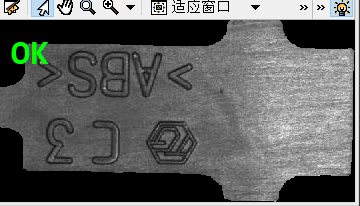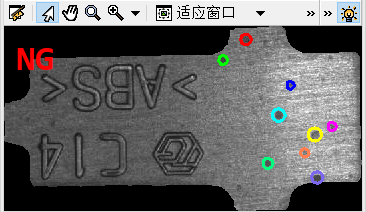展开全文• Bersen算法原理 　1） 先人为设定两个值S与TT(Bemsen最初设S为15，TT设为128)； 2）计算以图像中任意像素尸为中心的大小为k×k窗口内的所有像素的最大值M与最小值N，两者的均值T， 如果M-N大于S，则当前P的阈值...python 算法 计算机视觉 视觉检测 opencv
• 知道阈值分割的内容：全阈值分割，自适应阈值分割，熟悉大津法 知道分水岭算法的原理 了解GrabCut算法 1 图像分割 所谓图像分割指的是根据灰度、颜色、纹理和形状等特征把图像划分成若干互...人工智能 opencv
• ## 自适应阈值分割

千次阅读 2020-03-07 17:13:06
这种方法一般称为全局阈值分割，接下来想介绍的是一种局部阈值分割算法，其原理很简单，通俗地讲就是图片的每个局部都会通过处理得到一个阈值，这个区域就用这个阈值来进行分割，同理，每个区域都有不同的阈值来处理...opencv 算法
• ## 图像分割—灰度阈值分割

万次阅读 多人点赞 2019-08-14 11:39:55
阈值分割概念图像阈值分割具有直观和易于实现的特点，在图像分割应用中占有重要地位。许多情况下，图像$f(x,y)$由暗对象和亮对象这两类具有不同灰度级的区域组成，如报纸和书本。这种图像的亮暗部分可以在直方图中...数字图像处理 背景
• 常用的两种：全局阈值分割和自适应局部阈值分割。 全局阈值分割 全局阈值分割指的是将灰度值大于thresh（阈值）的图像设为白色，小于或者等于thresh的像素设为黑色；或者反只。 假设输入图像...opencv
• ## Halcon阈值分割

千次阅读 2018-12-30 10:41:38
Halcon阈值分割 1. threshold(Image : Region : MinGray, MaxGray : ) 全局阈值分割，适用于环境稳定，目标与背景存在明显的灰度差的场合。 应用1：利用灰度直方图确定阈值进行图像分割。一般是物体与背景之间...Halcon
• ## OpenCV阈值分割

千次阅读 2018-11-16 11:26:56
应用举例：从一副图像中利用阈值分割出我们需要的物体部分（当然这里的物体可以是一部分或者整体）。这样的图像分割方法是基于图像中物体与背景之间的灰度差异，而且此分割属于像素级的分割。 为了从一副图像中提取...
• ## 阈值分割法简述

千次阅读 2007-07-24 06:45:00
阈值分割法 阈值分割法分为全局阈值法和局部阈值分割法。所谓局部阈值分割法是将原始图像划分成较小的图像，并对每个子图像选取相应的阈值。在阈值分割后，相邻子图像之间的边界处可能产生灰度级的不连续性，因此...图像处理 算法
• 前面介绍了OTSU算法和最大熵算法，但这两种算法都属于全局阈值法，所以对于某些光照不均的图像，这种全局阈值分割的方法会显得苍白无力，如下图： 显然，这样的阈值处理结果不是我们想要的，那么就需要一种方法...
• ## otsu阈值分割

万次阅读 2018-11-06 21:03:18
一、OTSU法（大津阈值分割法）介绍   OTSU算法是由日本学者OTSU于1979年提出的一种对图像进行二值化的高效算法，是一种自适应的阈值确定的方法，又称大津阈值分割法，是最小二乘法意义下的最优分割。 二、单阈值...
• 针对各种阈值分割算法，本文在最后做了详细的研究，并给出了相应的MATLAB程序源代码。[关键词]：数字图像处理；图像分割；阈值；算法ResearchonlgorithmAbstract：ImageInthisthesis,thedigi...
• dyn_threshold(OrigImage, ThresholdImage : RegionDynThresh : Offset, LightDark : ) 对于一些目标与背景的阈值对比不明显的图像，需要用到动态阈值分割，使用局部阈值来分割图像。输出的图像...
• ## halcon阈值分割

千次阅读 2018-09-20 01:44:30
全局阈值分割，适用于环境稳定，目标与背景存在明显的灰度差的场合。 应用一 利用灰度直方图确定阈值进行图像分割。一般是物体与背景之间存在一个明显的灰度差，直方图会存在两个波峰一个是目标一个是背景，那么...
• 从大津法的原理上来讲，该方法又称作最大类间方差法，因为按照大津法求得的阈值进行图像二值化分割后，前景与背景图像的类间方差最大。 它被认为是图像分割阈值选取的最佳算法，计算简单，不受图像亮度和对比度的...opencv 计算机视觉
• 局部阈值分割自适应阈值 阈值的分割的核心就是如何选取阈值，选取正确的阈值时分割成功的关键。可以使用手动设置阈值，也可以采用直方图技术法、Otsu算法、熵算法自动选取全局阈值，也可以采用自适应阈值算法自动...opencv
• ## 阈值分割小结

千次阅读 2019-06-23 16:44:08
阈值分割 一： 全阈值分割 实例代码： image = cv2.imread('img.jpg', cv2.IMREAD_GRAYSCALE) the = 100 # 设置阈值为100 maxval = 255 dst, img = cv2.threshold(image, the, maxval, cv2.THRESH_BINARY) cv2....
• (1)进一步理解图像的阈值分割方法和边缘检测方法的原理。 (2)掌握图像基本全局阈值方法和最大类间方差法（otsu法）的原理并编程实现。 (3)编程实现图像的边缘检测。 二.实验内容和要求 编程实现图像阈值分割（基本...opencv 计算机视觉 数字图像处理
• 全局阈值分割` import numpy as np src = np.array([[123,234,68],[33,51,17],[48,98,234],[129,89,27],[45,167,134]]) src[src>150] = 255 src[src<=150] = 0 src 在图像处理中一般不处理原图 而是使用...图像分割
• ## 阈值分割介绍

千次阅读 2018-11-15 14:05:19
当人观察景物时，在视觉系统中对景物进行分割的过程是比不可少的，这个过程非常有效，以至于人所看到的并不是复杂的场景，而是一些物体的集合...图像的分割主要有：基于阈值分割方法、基于区域的分割方法、基于边...
• 自动全局阈值分割方法： 1、统计直方图 2、寻找出现频率最高的灰度值 3、把比最高频率灰度值或者比它大或小一定灰度阶的灰度值作为阈值分割图像 例： read_image(Image,'particle') gray_histo...halcon
• ## opencv学习-阈值分割

千次阅读 2019-06-06 18:41:56
把图像分成若干个特定的、具有独特性质的区域，每一个区域代表一个像素的集合，每一个集合代表一个物体，而完成该过程的技术通常称为图像...阈值分割处理主要是根据灰度值信息提取前景，对前景与背景有较强对比度...opencv
• 使用otsu大津法实现直方图的单阈值分割，可用于图像的分割。大津是用最大类间方差来判读阈值的，也可以用最小类内方差判读，最大类间方差+最小类内方差=总方差
• 当图像的色彩分布不均衡时，使用全局阈值处理的效果不是很好，这是使用局部阈值处理来进行分割，可以产生很好的效果。 局部阈值的处理原理是，针对每一个像素点专门配置一个阈值来进行处理，这些阈值就构成了和原图...opencv 计算机视觉
• 阈值分割基本原理 阈值分割因为具有简单、高效、实用的特点在图像分割领域得到了广泛的使用。阈值分割适用于灰度级存在明显差异情况下的前景背景分割。使用一个固定灰度值T作为阈值将前景背景区域进行分割，遍历整幅...计算机视觉
•机器视觉...